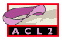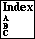## REWRITE

make some `:rewrite` rules (possibly conditional ones)
```Major Section:  RULE-CLASSES
```

See rule-classes for a general discussion of rule classes and how they are used to build rules from formulas. Example `:``corollary` formulas from which `:rewrite` rules might be built are:

```Example:
(equal (+ x y) (+ y x))            replace (+ a b) by (+ b a) provided
certain heuristics approve the
permutation.

(implies (true-listp x)            replace (append a nil) by a, if
(equal (append x nil) x)) (true-listp a) rewrites to t

(implies                           replace (member a (append b c)) by
(and (eqlablep e)              (member a (append c b)) in contexts
(true-listp x)            in which propositional equivalence
(true-listp y))           is sufficient, provided (eqlablep a)
(iff (member e (append x y))   (true-listp b) and (true-listp c)
(member e (append y x)))) rewrite to t and the permutative
heuristics approve

General Form:
(and ...
(implies (and ...hi...)
(implies (and ...hk...)
(and ...
(equiv lhs rhs)
...)))
...)
```
Note: One `:rewrite` rule class object might create many rewrite rules from the `:``corollary` formula. To create the rules, we first translate the formula (expanding all macros; also see trans). Next, we eliminate all `lambda`s; one may think of this step as simply substituting away every `let`, `let*`, and `mv-let` in the formula. We then flatten the `and` and `implies` structure of the formula, transforming it into a conjunction of formulas, each of the form
```(implies (and h1 ... hn) concl)
```
where no hypothesis is a conjunction and `concl` is neither a conjunction nor an implication. If necessary, the hypothesis of such a conjunct may be vacuous. We then further coerce each `concl` into the form `(equiv lhs rhs)`, where `equiv` is a known equivalence relation, by replacing any `concl` not of that form by `(iff concl t)`. A `concl` of the form `(not term)` is considered to be of the form `(iff term nil)`. By these steps we reduce the given `:``corollary` to a sequence of conjuncts, each of which is of the form
```(implies (and h1 ... hn)
(equiv lhs rhs))
```
where `equiv` is a known equivalence relation. See equivalence for a general discussion of the introduction of new equivalence relations.

We create a `:rewrite` rule for each such conjunct, if possible, and otherwise cause an error. It is possible to create a rewrite rule from such a conjunct provided `lhs` is not a variable, a quoted constant, a `let`-expression, a `lambda` application, or an `if`-expression.

A `:rewrite` rule is used when any instance of the `lhs` occurs in a context in which the equivalence relation is operative. First, we find a substitution that makes `lhs` equal to the target term. Then we attempt to relieve the instantiated hypotheses of the rule. Hypotheses that are fully instantiated are relieved by recursive rewriting. Hypotheses that contain ``free variables'' (variables not assigned by the unifying substitution) are relieved by attempting to guess a suitable instance so as to make the hypothesis equal to some known assumption in the context of the target. If the hypotheses are relieved, and certain restrictions that prevent some forms of infinite regress are met (see loop-stopper), the target is replaced by the instantiated `rhs`, which is then recursively rewritten.

ACL2's rewriting process has undergone some optimization. In particular, when a term `t1` is rewritten to a new term `t2`, the rewriter is then immediately applied to `t2`. On rare occasions you may find that you do not want this behavior, in which case you may wish to use a trick involving `hide` that is described near the end of the documentation for meta; see meta.

At the moment, the best description of how ACL2 `:rewrite` rules are used is perhaps in the discussion of ``Replacement Rules'' on page 279 of A Computational Logic Handbook (second edition).